# NCERT Class 10: Science-Intext Solutions

### Chapter 12-Electricity-Intext Solutions

NCERT Book Page Number- 200
Q.1. What does an electric circuit mean?
Ans. A continuous and closed path of an electric current is called an electric circuit. An electric circuit consist of electric devices, source of electricity and wires that are connected with help of a switch.
Q.2. Define the unit of current.
Ans. SI unit of electric current is ampere (A). If 1 coulomb of electric charge flows through a cross-section of wire for 1 second, then it would be equal to 1 ampere. So, 1 ampere = 1C /1s i.e. 1 A = 1 C/s
Q.3. Calculate the number of electrons constituting one coulomb of charge.
Ans. One electron possesses a charge of $1.6 × 10^{-19} C$
Given q = 1 C, e = $1.6 × 10^{-19} C$
n = ?
q = ne
n = q/e
n = 1 C /$1.6 × 10^{-19} C$= $6.25 × 10^{18}$ electrons.

NCERT Book Page Number- 202
Q.1. Name a device that helps to maintain a potential difference across a conductor.
Ans. Any source of electricity like battery, cell, power supply, etc. help to maintain a potential difference across a conductor.
Q.2. What is meant by saying that the potential difference between two points is 1 V?
Ans. If 1 J of work is required to move a charge of amount 1 C from one point to another, then it is said that the potential difference between the two points is 1 V.
Q.3. How much energy is given to each coulomb of charge passing through a 6 V battery?
Ans. The energy given to each coulomb of charge is equal to the amount of work which is done in moving it.
Now we know that,
Potential difference = Work done Charge
∴ Work done = Potential difference × Charge
Charge = 1 C and Potential difference = 6 V (given)
∴ Work done = 6 × 1 = 6 J

NCERT Book Page Number- 209

Q.1. On what factors does the resistance of a conductor depend?
Ans. The resistance of a conductor depends upon the following factors.
(i) Length of the conductor. (ii) Cross-sectional area of the conductor.
(iii) Material of the conductor. (iv) Temperature of the conductor.
Q.2. Will current flow more easily through a thick wire or a thin wire of the same material, when connected to the same source? Why?
Ans. The current will flow more easily through thick wire. It is because the resistance of a conductor is inversely proportional to its area of cross-section. If thicker the wire, less is resistance and hence more easily the current flows.
Q.3. Let the resistance of an electrical component remains constant while the potential difference across the two ends of the component decreases to half of its former value. What change will occur in the current through it?
Ans. According to Ohm’s law
V = IR ⇒ I = VR …(1)
Now Potential difference is decreased to half.
∴ New potential difference V’ = V/2
Resistance remains constant.
from Ohm’s law I = V/R
So, the new current I′ = V′/ R
⇒ I′ = V/2R
⇒ I = 1/2 × VR
⇒ I′ = 1/2 × I = I/2
Therefore, the amount of current flowing through the electrical component is reduced by half.
Q.4. Why are coils of electric toasters and electric irons made of an alloy rather than a pure metal?
Ans. The resistivity of an alloy is higher than the pure metal. Moreover, at high temperatures, the alloy do not melt readily. Hence, the coils of heating appliances such as electric toasters and electric irons are made of an alloy than a pure metal.
Q.5. Use the data in Table 1.1 to answer the following:
(a) Which among iron and mercury is a better conductor?
(b) Which material is the best conductor?
Table 1.1 Electrical resistivity of some substance at 20°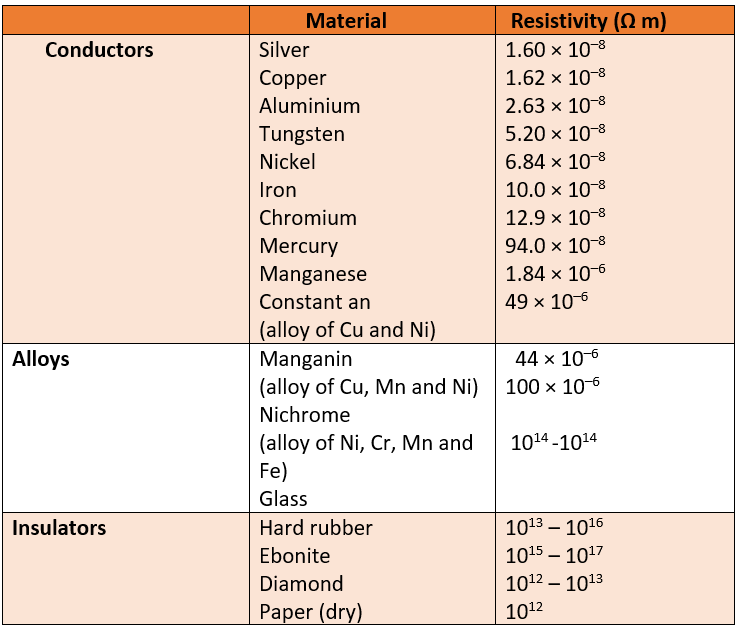Ans. (a) Resistivity of iron = $10.0 × 10^{-8} Ω$

Resistivity of mercury = $94.0 × 10^{-8} Ω$
Resistivity of mercury is more than that of iron. This implies that iron is a better conductor than mercury.
(b) It can be observed from Table 1.1 that the resistivity of silver is the lowest among the listed materials. Hence, it is the best conductor.

NCERT Book Page Number- 213
Q.1. Draw a schematic diagram of a circuit consisting of a battery of three cells of 2 V each, a 5 Ω resistor, an 8 Ω resistor, and a 12 Ω resistor, and a plug key, all connected in series.
Ans. Three cells of potential 2 V, each connected in series therefore the potential difference of the battery will be 2 V + 2 V + 2 V = 6 V. The following circuit diagram shows three resistors of resistances 5 Ω, 8 Ω and 12 Ω respectively connected in series and a battery of potential 6 V and a plug key which is closed means the current is flowing in the circuit.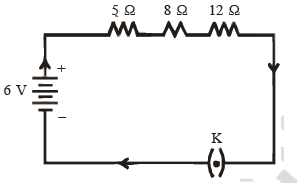Q.2. Redraw the circuit of question 12, putting in an ammeter to measure the current through the resistors and a voltmeter to measure potential difference across the 12 Ω resistor. What would be the readings in the ammeter and the voltmeter?
Ans. An ammeter should be connected in the circuit in series with the resistors. To measure the potential difference across the resistor it should be connected in parallel, as shown in the following figure.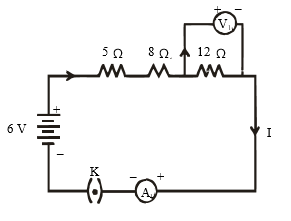The resistances are connected in series.
Ohm’s law can be used to obtain the readings of ammeter and voltmeter. According to Ohm’s law,
V = IR,
where,
Potential difference, V = 6 V
Current flowing through the circuit (resistors) = I
Resistance of the circuit,

R = 5 + 8 + 12 = 25 Ω
I = V/R = 6/25 = 0.24 A

Potential difference across 12 Ω restore = V1
Current flowing through the 12 Ω resistor, I = 0.24 A
Therefore, using Ohm’s law, we obtain
$V_{1}$ = IR = 0.24 × 12 = 2.88 V

Therefore, the reading of the ammeter will be 0.24 A.
The reading of the voltmeter will be 2.88 V.

NCERT Book Page Number- 216
Q.1. Judge the equivalent resistance when the following are connected in parallel :
(a) $1 Ω and 10^{6} Ω, (b) 1 Ω and 10^{3} Ω and 10^{6} Ω.$
Ans. (a) When 1 Ω and $10^{6} Ω$ are connected in parallel:
Let R be the equivalent resistance.
Equivalent resistance in parallel combination of resistors is always less than the least resistance of any resistors in the circuit.
Hence, in both the cases i.e., (a) and (b) the equivalent resistance is less than 1Ω.
Q.2. An electric lamp of 100 Ω, a toaster of resistance 50 Ω and a water filter of resistance 500 Ω are connected in parallel to a 220 V source. What is the resistance of an electric iron to the same source that takes as much current as all three appliances, and what is the current through it?
Ans. Resistance of electric lamp, $R_{1}$ = 100 Ω
Resistance of toaster,$R_{2}$ = 50 Ω
Resistance of water filter, $R_{3}$ = 500 Ω
Potential difference of the source,
V = 220 V
These appliances are connected in parallel.
Let R be the equivalent resistance of the circuit.
then, 1/R = 1/$R_{1}$ + 1/$R_{2}$ + 1/$R_{3}$ = 1/100 + 1/50 + 1/500
= (5 +10 +1)/500
⇒ 1/R = 16/ 500
R = 500/16 Ω
According to Ohm’s law,
V = IR
I = V/R
where, Current flowing through the circuit = I
I = 220 × 16/ 500 = = 7.04 A
7.04 A of current is drawn by all the three given appliances.
Therefore, current drawn by an electric iron connected to the same source of potential 220 V = 7.04 A
Let R′ be the resistance of the electric iron. According to Ohm’s law,
V = IR′

R′ = V/I = 220 /7.04

Therefore, the resistance of the electric iron is 31.25 Ω and the current flowing through it is 7.04 A.
Q.3. What are the advantages of connecting electrical devices in parallel with the battery instead of connecting them in series?
Ans. Advantage of connecting electrical devices in parallel:
(i) When the appliances are connected in parallel with the battery, each appliance gets the same potential difference as that of battery which is not possible in series connection.
(ii) Each appliance has different resistances and requires different currents to operate properly. This is possible only in parallel connection, as in series connection, same current flows through all devices, irrespective of their resistances.
(iii) If one appliance fails to work, other will continue to work property. If they are connected in parallel.
Q.4. How can three resistors of resistances 2 Ω, 3 Ω and 6 Ω be connected to give a total resistance of (a) 4 Ω, (b) 1 Ω?
Ans. There are three resistors of resistances 2 Ω, 3 Ω and 6 Ω respectively.
(a) The following circuit diagram shows the connection of the three resistors.
Here, 6 Ω and 3 Ω resistors are connected in parallel.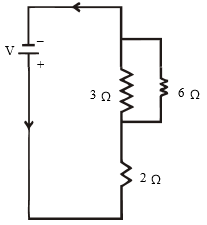Therefore, their equivalent resistance will be given by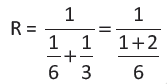This equivalent resistor of resistance 2 Ω is connected to a 2 Ω resistor in series.
Therefore, the equivalent resistance of the circuit = 2 Ω + 2 Ω = 4 Ω
Hence the total resistance of the circuit is 4 Ω.
(b) The following circuit diagram shows the connection of the three resistors.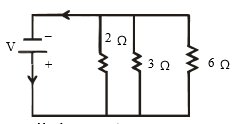All the resistors are connected in parallel. Therefore, their equivalent resistance will be given as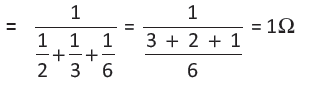Therefore, the total resistance of the circuit is 1 Ω.

Q.5. What is (a) the highest, (b) the lowest total resistance that can be secured by combinations of four coils of resistance 4 Ω, 8 Ω, 12 Ω, 24 Ω ?
Ans. There are four coils of resistances 4 Ω, 8 Ω, 12 Ω and 24 Ω respectively.
(a) If these coils are connected in series, then the equivalent resistance will be the highest, given by the sum 4 + 8 + 12 + 24 = 48 Ω.
(b) If these coils are connected in parallel, then the equivalent resistance will be the lowest, given by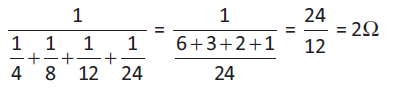Therefore, 2 Ω is the lowest total resistance.

NCERT Book Page Number- 218
Q.1. Why does the cord of an electric heater not glow while the heating element does?
Ans. The heating element of the heater is made up of alloy which has very high resistance so when current flows through the heating element, it becomes too hot and glows red. But the resistance of cord which is usually of copper or aluminium is very low as compared to heating element, so it does not glow.
Q.2. Compute the heat generated while transferring 96000 coulomb of charge in one hour through a potential difference of 50 V.
Ans. Given charge, Q = 96000 C
Time, t = 1 hr = 60 × 60 = 3600 s
Potential difference, V = 50 volts
Now we know that H = VIt
So we have to calculate, I first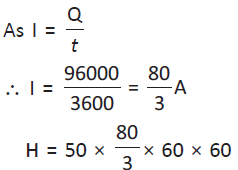$= 4.8 × 10_{6} J$
Therefore, the heat generated is $4.8 × 10_{6} J.$
Q.3. An electric iron of resistance 20 Ω takes a current of 5 A. Calculate the heat developed in 30 s.
Ans. The amount of heat (H) produced is given by the Joule’s law of heating as H = VIt
where, Current, I = 5 A
Time, t = 30 s
Voltage, V = Current × Resistance = 5 × 20 = 100 V

H = 100 × 5 × 30 = $1.5 × 10_{4}$ J.
Therefore, the amount of heat developed in the electric iron is $1.5 × 10_{4}$ J.

NCERT Book Page Number- 220

Q.1. What determines the rate at which energy is delivered by a current?
Ans. The rate of consumption of electric energy in an electric appliance is called electric power.
Hence, the rate at which energy is delivered by a current is the power of the appliance.
Q.2. An electric motor takes 5 A from a 220 V line. Determine the power of the motor and the energy consumed in 2 h.
Ans. Power (P) is given by the expression, P = VI
where, Voltage, V = 220 V
Current, I = 5 A
P = 220 × 5 = 1100 W
Energy consumed by the motor = Pt
where, Time, t = 2 h = 2 × 60 × 60 = 7200 s
∴ P = 1100 × 7200 = 7.92 × 106 J
Therefore, power of the motor = 1100 W
Energy consumed by the motor = $7.92 × 10^{6} J.$